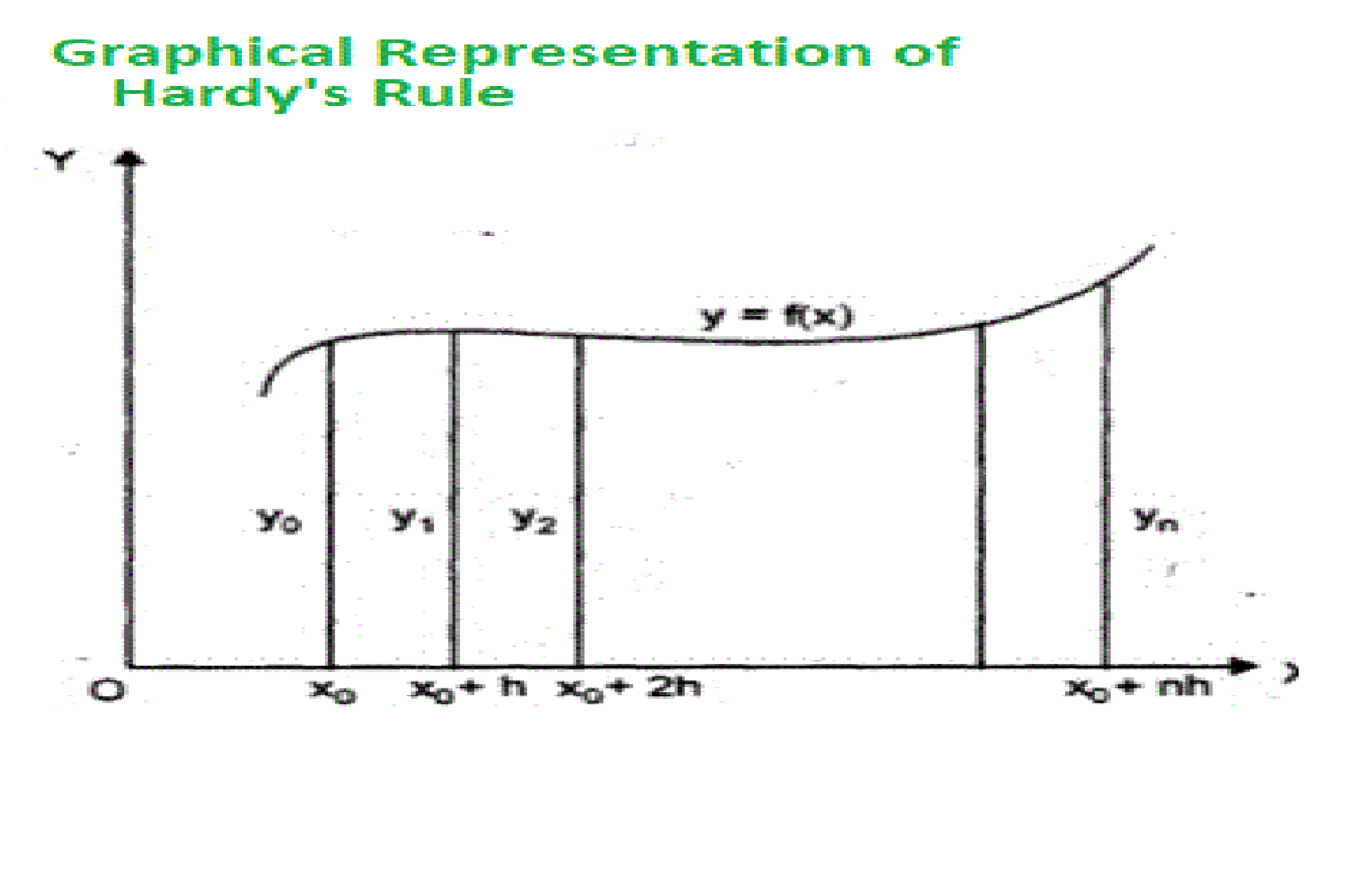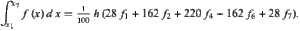# Hardy’s Rule

Hardy’s Rule is an extension of Newton–Cotes formulas. Consider a function, f(x), tabulated at pointsequally spaced bysuch thatGiven the following inputs
1. A function, whose integrand has to be computed.
2. The upper and lower limitsHardy’s rule can be derived by approximating the integrand f(x)

Example :

The task is to find the integrand of the function using Hardy’s Rule

```upper limit, b = 6,
lower limit a = 0 .
```

Approach :
Hardy’s Rule is a numerical integration technique to find the approximate value of the integral.

``````are the values of f(x) at their respective intervals of x.
In order to integrate any function f(x) in the interval (a, b), follow the steps given below:

1.the value of n=6, which is the number of parts the interval is divided into.
2.Calculate the width, h = (b-a)/6
3.Calculate the values of x0 to x6 asConsider y = f(x). Now find the values offor the correspondingvalues.
4. Substitute all the above-found values in the Hardy’s rule to calculate the integral value.

Below is the implementation of the above approach:

 `// C program to implement Hardy's Rule ` `// on the given function ` ` `  `#include ` `#include ` ` `  `// In order to represent the implementation, ` `// a function f(x) = 1/(1 + x) is considered ` `// in this program ` ` `  `// Function to return the value of f(x) ` `// for the given value of x ` `float` `y(``float` `x) ` `{ ` `    ``return` `(1 / (1 + x)); ` `} ` ` `  `// Function to computes the integrand of y ` `// at the given intervals of x with ` `// step size h and the initial limit a ` `// and final limit b ` `float` `Hardyrule(``float` `a, ``float` `b) ` `{ ` `    ``// Number of intervals ` ` `  `    ``int` `n = 6; ` `    ``int` `h; ` ` `  `    ``// Computing the step size ` `    ``h = ((b - a) / n); ` `    ``float` `sum = 0; ` ` `  `    ``// Substituing a = 0, b = 4 and h = 1 ` `    ``float` `hl = (28* y(a) + 162 * y(a + h) ` ` `  `                ``+ 220 * y(a + 3 * h) ` `                ``+  162* y(a + 5 * h) ` `                ``+28* y(a + 6*h))*h/100 ` `                ``; ` ` `  `    ``sum = sum + hl; ` `    ``return` `sum; ` `} ` ` `  `// Driver code ` `int` `main() ` `{ ` `    ``float` `lowlimit = 0; ` `    ``float` `upplimit = 6; ` `    ``printf``(``"f(x) = %.4f"``, ` `           ``Hardyrule(0, 6)); ` `    ``return` `0; ` `} `

Output:

```f(x) = 1.9500
```

Don’t stop now and take your learning to the next level. Learn all the important concepts of Data Structures and Algorithms with the help of the most trusted course: DSA Self Paced. Become industry ready at a student-friendly price.

My Personal Notes arrow_drop_upCheck out this Author's contributed articles.

If you like GeeksforGeeks and would like to contribute, you can also write an article using contribute.geeksforgeeks.org or mail your article to contribute@geeksforgeeks.org. See your article appearing on the GeeksforGeeks main page and help other Geeks.

Please Improve this article if you find anything incorrect by clicking on the "Improve Article" button below.

Article Tags :

Be the First to upvote.

Please write to us at contribute@geeksforgeeks.org to report any issue with the above content.